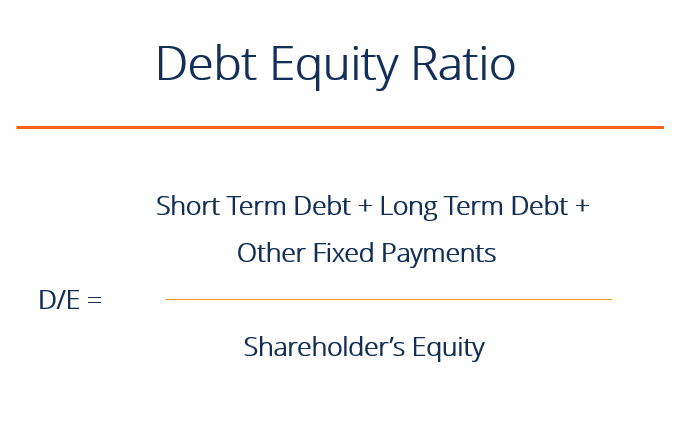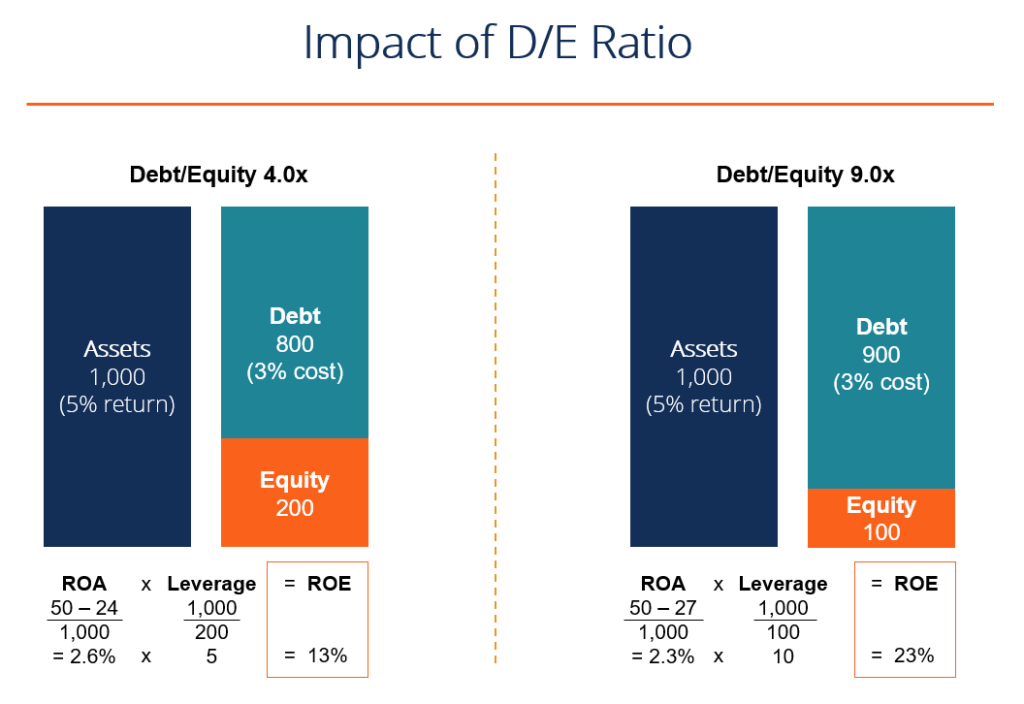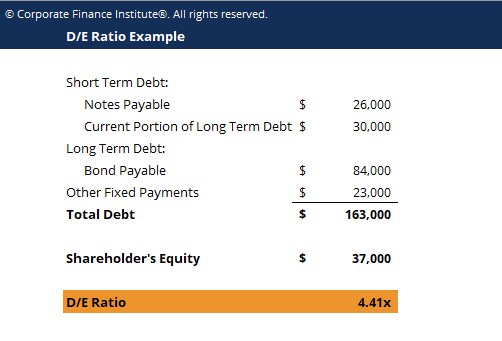# Debt Equity Ratio

How much leverage does a company have?

## What is the Debt to Equity Ratio?

The Debt to Equity ratio (also called the “debt-equity ratio”, “risk ratio”, or “gearing”), is a leverage ratio that calculates the weight of total debt and financial liabilities against the total shareholders’ equity. Unlike the debt-assets ratio which uses total assets as a denominator, the D/E Ratio uses total equity. This ratio highlights how a company’s capital structure is tilted either toward debt or equity financing.### Debt to Equity Ratio Formula

Short formula:

Debt to Equity Ratio = Total Debt / Shareholders’ Equity

Long formula:

Debt to Equity Ratio = (short term debt + long term debt + fixed payment obligations) / Shareholders’ Equity

### Debt to Equity Ratio in Practice

If, as per the balance sheet, the total debt of a business is worth \$50 million and the total equity is worth \$120 million, then debt-to-equity is 0.42. This means that for every dollar in equity, the firm has 42 cents in leverage. A ratio of 1 would imply that creditors and investors are on equal footing in the company’s assets.

A higher debt-equity ratio indicates a levered firm, which is quite preferable for a company that is stable with significant cash flow generation, but not preferable when a company is in decline. Conversely, a lower ratio indicates a firm less levered and closer to being fully equity financed. The appropriate debt to equity ratio varies by industry.

Learn all about calculating leverage ratios step by step in CFI’s Financial Analysis Fundamentals Course!

### What is Total Debt?

A company’s total debt is the sum of short-term debt, long-term debt, and other fixed payment obligations (such as capital leases) of a business that are incurred while under normal operating cycles. Creating a debt schedule helps split out liabilities by specific pieces.

Not all current and non-current liabilities are considered debt.  Below are some examples of things that are and are not considered debt.

Considered debt:

• Drawn line-of-credit
• Notes payable (maturity within a year)
• Current portion of Long-Term Debt
• Notes payable (maturity more than a year)
• Bonds payable
• Long-Term Debt
• Capital lease obligations

Not considered debt:

• Accounts payable
• Accrued expenses
• Deferred revenues
• Dividends payable

### Benefits of a High D/E Ratio

A high debt-equity ratio can be good because it shows that a firm can easily service its debt obligations (through cash flow) and is using the leverage to increase equity returns.

In the example below, we see how using more debt (increasing the debt-equity ratio) increases the company’s return on equity (ROE).  By using debt instead of equity, the equity account is smaller and therefore, return on equity is higher.

Another benefit is that typically the cost of debt is lower than the cost of equity, and therefore increasing the D/E ratio (up to a certain point) can lower a firm’s weighted average cost of capital (WACC).The topic above is covered in more detail in CFI’s Free Corporate Finance Course!

### Drawbacks of a High D/E Ratio

The opposite of the above example applies if a company has a D/E ratio that’s too high.  In this case, any losses will be compounded down and the company may not be able to service its debt.

If the debt to equity ratio gets too high, the cost of borrowing will skyrocket, as will the cost of equity, and the company’s WACC will get extremely high, driving down its share price.

### Debt to Equity Ratio Calculator

Below is a simple example of an Excel calculator to download and see how the number works on your own.### Video Explanation of the Debt to Equity Ratio

Below is a short video tutorial that explains how leverage impacts a company and how to calculate the debt/equity ratio with an example.

Video: CFI’s Financial Analysis Courses

### More Resources

Thank you for reading CFI’s guide to the debt to equity ratio. CFI is the official provider of the Financial Modeling and Valuation Analyst (FMVA)® designation designed to transform anyone into a world-class financial analyst.

To keep learning and developing your knowledge of financial analysis we highly recommend these additional resources:

• Analysis of Financial Statements
• Price Earnings Ratio
• EV/EBITDA Ratio
• Financial Modeling Guide

### Financial Analyst Training

Get world-class financial training with CFI’s online certified financial analyst training program!

Gain the confidence you need to move up the ladder in a high powered corporate finance career path.

Learn financial modeling and valuation in Excel the easy way, with step-by-step training.